xJordan normal formEncyclopedia
In linear algebra
Linear algebra
Linear algebra is a branch of mathematics that studies vector spaces, also called linear spaces, along with linear functions that input one vector and output another. Such functions are called linear maps and can be represented by matrices if a basis is given. Thus matrix theory is often...

, a Jordan normal form (often called Jordan canonical form)
of a linear operator on a finite-dimensional vector space
Vector space
A vector space is a mathematical structure formed by a collection of vectors: objects that may be added together and multiplied by numbers, called scalars in this context. Scalars are often taken to be real numbers, but one may also consider vector spaces with scalar multiplication by complex...

is an upper triangular matrix of a particular form called Jordan matrix
Jordan matrix
In the mathematical discipline of matrix theory, a Jordan block over a ring R is a matrix composed of 0 elements everywhere except for the diagonal, which is filled with a fixed element \lambda\in R, and for the superdiagonal, which is composed of ones...

, representing the operator on some basis
Basis (linear algebra)
In linear algebra, a basis is a set of linearly independent vectors that, in a linear combination, can represent every vector in a given vector space or free module, or, more simply put, which define a "coordinate system"...

. The form is characterized by the condition that any non-diagonal entries that are non-zero must be equal to 1, be immediately above the main diagonal (on the superdiagonal), and have identical diagonal entries to the left and below them. If the vector space is over a field
Field (mathematics)
In abstract algebra, a field is a commutative ring whose nonzero elements form a group under multiplication. As such it is an algebraic structure with notions of addition, subtraction, multiplication, and division, satisfying certain axioms...

K, then a basis on which the matrix has the required form exists if and only if
If and only if
In logic and related fields such as mathematics and philosophy, if and only if is a biconditional logical connective between statements....

all eigenvalues of M lie in K, or equivalently if the characteristic polynomial
Characteristic polynomial
In linear algebra, one associates a polynomial to every square matrix: its characteristic polynomial. This polynomial encodes several important properties of the matrix, most notably its eigenvalues, its determinant and its trace....

of the operator splits into linear factors over K. This condition is always satisfied if K is the field of complex number
Complex number
A complex number is a number consisting of a real part and an imaginary part. Complex numbers extend the idea of the one-dimensional number line to the two-dimensional complex plane by using the number line for the real part and adding a vertical axis to plot the imaginary part...

s. The diagonal entries of the normal form are the eigenvalues of the operator, with the number of times each one occurs being given by its algebraic multiplicity.

If the operator is originally given by a square matrix M, then its Jordan normal form is also called the Jordan normal form of M. Any square matrix has a Jordan normal form if the field of coefficients is extended to one containing all the eigenvalues of the matrix. In spite of its name, the normal form for a given M is not entirely unique, as it is a block diagonal matrix formed of Jordan blocks, the order of which is not fixed; it is conventional to group blocks for the same eigenvalue together, but no ordering is imposed among the eigenvalues, nor among the blocks for a given eigenvalue, although the latter could for instance be ordered by weakly decreasing size. The Jordan–Chevalley decomposition is particularly simple on a basis on which the operator takes its Jordan normal form. The diagonal form for diagonalizable matrices, for instance normal matrices
Normal matrix
A complex square matrix A is a normal matrix ifA^*A=AA^* \ where A* is the conjugate transpose of A. That is, a matrix is normal if it commutes with its conjugate transpose.If A is a real matrix, then A*=AT...

, is a special case of the Jordan normal form.

The Jordan normal form is named after Camille Jordan
Camille Jordan
Marie Ennemond Camille Jordan was a French mathematician, known both for his foundational work in group theory and for his influential Cours d'analyse. He was born in Lyon and educated at the École polytechnique...

.

## Motivation

An n × n matrix A is diagonalizable
Diagonalizable matrix
In linear algebra, a square matrix A is called diagonalizable if it is similar to a diagonal matrix, i.e., if there exists an invertible matrix P such that P −1AP is a diagonal matrix...

if and only if the sum of the dimensions of the eigenspaces is n. Or, equivalently, if and only if A has n linearly independent eigenvectors. Not all matrices are diagonalizable. Consider the following matrix:Including multiplicity, the eigenvalues of A are λ = 1, 2, 4, 4. The dimension of the kernel of (A − 4In
Identity matrix
In linear algebra, the identity matrix or unit matrix of size n is the n×n square matrix with ones on the main diagonal and zeros elsewhere. It is denoted by In, or simply by I if the size is immaterial or can be trivially determined by the context...

) is 1 (and not 2), so A is not diagonalizable. However, there is an invertible matrix P such that A = PJP−1, whereThe matrix J is almost diagonal. This is the Jordan normal form of A. The section Example below fills in the details of the computation.

## Complex matrices

In general, a square complex matrix A is similar to a block diagonal matrix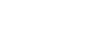where each block Ji is a square matrix of the form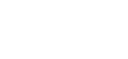So there exists an invertible matrix P such that P-1AP = J is such that the only non-zero entries of J are on the diagonal and the superdiagonal. J is called the Jordan normal form of A. Each Ji is called a Jordan block of A. In a given Jordan block, every entry on the super-diagonal is 1.

Assuming this result, we can deduce the following properties:
• Counting multiplicity, the eigenvalues of J, therefore A, are the diagonal entries.

• Given an eigenvalue λi, its geometric multiplicity is the dimension of Ker(A − λi I
Identity matrix
In linear algebra, the identity matrix or unit matrix of size n is the n×n square matrix with ones on the main diagonal and zeros elsewhere. It is denoted by In, or simply by I if the size is immaterial or can be trivially determined by the context...

), and it is the number of Jordan blocks corresponding to λi.

• The sum of the sizes of all Jordan blocks corresponding to an eigenvalue λi is its algebraic multiplicity.

• A is diagonalizable if and only if, for every eigenvalue λ of A, its geometric and algebraic multiplicities coincide.

• The Jordan block corresponding to λ is of the form λ I + N, where N is a nilpotent matrix
Nilpotent matrix
In linear algebra, a nilpotent matrix is a square matrix N such thatN^k = 0\,for some positive integer k. The smallest such k is sometimes called the degree of N....

defined as Nij = δi,j−1 (where δ is the Kronecker delta). The nilpotency of N can be exploited when calculating f(A) where f is a complex analytic function. For example, in principle the Jordan form could give a closed-form expression for the exponential exp(A).

### Generalized eigenvectors

Consider the matrix A from the example in the previous section. The Jordan normal form is obtained by some similarity transformation P−1AP = J, i.e.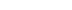Let P have column vectors pi, i = 1, ..., 4, then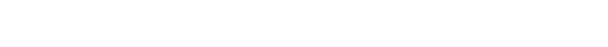We see that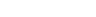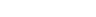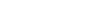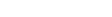For i = 1,2,3 we have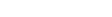, i.e. pi is an eigenvector of A corresponding to the eigenvalue λi. For i=4, multiplying both sides by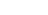gives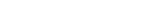But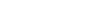, so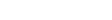Thus,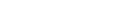.

Vectors such as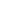are called generalized eigenvector
Generalized eigenvector
In linear algebra, for a matrix A, there may not always exist a full set of linearly independent eigenvectors that form a complete basis – a matrix may not be diagonalizable. This happens when the algebraic multiplicity of at least one eigenvalue λ is greater than its geometric multiplicity...

s of A.

Thus, given an eigenvalue λ, its corresponding Jordan block gives rise to a Jordan chain. The generator, or lead vector, say pr, of the chain is a generalized eigenvector such that (A − λ I)rpr = 0, where r is the size of the Jordan block. The vector p1 = (A − λ I)r−1pr is an eigenvector corresponding to λ. In general, pi is a preimage of pi−1 under A − λ I. So the lead vector generates the chain via multiplication by (A − λ I).

Therefore, the statement that every square matrix A can be put in Jordan normal form is equivalent to the claim that there exists a basis consisting only of eigenvectors and generalized eigenvectors of A.

### A proof

We give a proof by induction. The 1 × 1 case is trivial. Let A be an n × n matrix. Take any eigenvalue λ of A. The range of A − λ I, denoted by Ran(A − λ I), is an invariant subspace
Invariant subspace
In mathematics, an invariant subspace of a linear mappingfrom some vector space V to itself is a subspace W of V such that T is contained in W...

of A. Also, since λ is an eigenvalue of A, the dimension Ran(A − λ I), r, is strictly less than n. Let A' denote the restriction of A to Ran(A − λ I), By inductive hypothesis, there exists a basis {p1, ..., pr} such that A' , expressed in terms of this basis, is in Jordan normal form.

Next consider the subspace Ker(A − λ I). If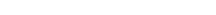the desired result follows immediately from the rank–nullity theorem. This would be the case, for example, if A was Hermitian.

Otherwise, if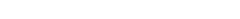let the dimension of Q be sr. Each vector in Q is an eigenvector of A' corresponding to eigenvalue λ. So the Jordan form of A' must contain s Jordan chains corresponding to s linearly independent eigenvectors. So the basis {p1, ..., pr} must contain s vectors, say {prs+1, ..., pr}, that are lead vectors in these Jordan chains from the Jordan normal form of A. We can "extend the chains" by taking the preimages of these lead vectors. (This is the key step of argument; in general, generalized eigenvectors need not lie in Ran(A − λ I).) Let qi be such that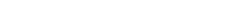Clearly
qi does not lie in Ker(A − λ I) for all i. Furthermore, qi cannot be in Ran(A − λ
I), for that would contradict the assumption that each pi is a lead vector in a Jordan chain. The set {qi}, being preimages of the linearly independent set {pi} under A − λ I, is also linearly independent.

Finally, we can pick any linearly independent set {
z1, ..., zt} that spans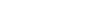By construction, the union the three sets {
p1, ..., pr}, {qrs +1, ..., qr}, and {z1, ..., zt} is linearly independent. Each vector in the union is either an eigenvector or a generalized eigenvector of A. Finally, by rank–nullity theorem, the cardinality of the union is n. In other words, we have found a basis that consists of eigenvectors and generalized eigenvectors of A, and this shows A can be put in Jordan normal form.

### Uniqueness

It can be shown that the Jordan normal form of a given matrix
A is unique up to the order of the Jordan blocks.

Knowing the algebraic and geometric multiplicities of the eigenvalues is not sufficient to determine the Jordan normal form of
A. Assuming the algebraic multiplicity m(λ) of an eigenvalue λ is known, the structure of the Jordan form can be ascertained by analysing the ranks of the powers (A − λ I)m(λ). To see this, suppose an n × n matrix A has only one eigenvalue λ. So m(λ) = n. The smallest integer k1 such that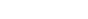is the size of the largest Jordan block in the Jordan form of
A. (This number k1 is also called the index of λ. See discussion in a following section.) The rank of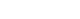is the number of Jordan blocks of size
k1. Similarly, the rank of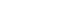is twice the number of Jordan blocks of size
k1 plus the number of Jordan blocks of size k1 − 1. Iterating in this way gives the precise Jordan structure of A. The general case is similar.

This can be used to show the uniqueness of the Jordan form. Let
J1 and J2 be two Jordan normal forms of A. Then J1 and J2 are similar and have the same spectrum, including algebraic multiplicities of the eigenvalues. The procedure outlined in the previous paragraph can be used to determine the structure of these matrices. Since the rank of a matrix is preserved by similarity transformation, there is a bijection between the Jordan blocks of J1 and J2. This proves the uniqueness part of the statement.

## Real matrices

If
A is a real matrix, its Jordan form can still be non-real, however there exists a real invertible matrix P such that P-1AP = J is a real block diagonal matrix with each block being a real Jordan block. A real Jordan block is either identical to a complex Jordan block (if the corresponding eigenvalue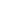is real), or is a block matrix itself, consisting of 2×2 blocks as follows (for non-real eigenvalue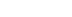). The diagonal blocks are identical, of the form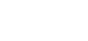and describe multiplication byin the complex plane. The superdiagonal blocks are 2×2 identity matrices. The full real Jordan block is given by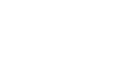This real Jordan form is a consequence of the complex Jordan form. For a real matrix the non real eigenvectors and generalized eigenvectors can always be chosen to form complex conjugate
Complex conjugate
In mathematics, complex conjugates are a pair of complex numbers, both having the same real part, but with imaginary parts of equal magnitude and opposite signs...

pairs. Taking the real and imaginary part (linear combination of the vector and its conjugate), the matrix has this form in the new basis.

## Consequences

One can see that the Jordan normal form is essentially a classification result for square matrices, and as such several important results from linear algebra can be viewed as its consequences.

### Spectral mapping theorem

Using the Jordan normal form, direct calculation gives a spectral mapping theorem for the polynomial functional calculus
Functional calculus
In mathematics, a functional calculus is a theory allowing one to apply mathematical functions to mathematical operators. It is now a branch of the field of functional analysis, connected with spectral theory. In mathematics, a functional calculus is a theory allowing one to apply mathematical...

: Let A be an n × n matrix with eigenvalues λ1, ..., λn, then for any polynomial p, p(A) has eigenvalues p1), ..., pn).

### Cayley–Hamilton theorem

The Cayley–Hamilton theorem
Cayley–Hamilton theorem
In linear algebra, the Cayley–Hamilton theorem states that every square matrix over a commutative ring satisfies its own characteristic equation....

asserts that every matrix
A satisfies its characteristic equation: if is the characteristic polynomial
Characteristic polynomial
In linear algebra, one associates a polynomial to every square matrix: its characteristic polynomial. This polynomial encodes several important properties of the matrix, most notably its eigenvalues, its determinant and its trace....

of , then . This can be shown via direct calculation in the Jordan form, since any Jordan block for is annihilated by where is the multiplicity of the root of , the sum of the sizes of the Jordan blocks for , and therefore no less than the size of the block in question. The Jordan form can be assumed to exist over a field extending the base field of the matrix, for instance over the splitting field
Splitting field
In abstract algebra, a splitting field of a polynomial with coefficients in a field is a smallest field extension of that field over which the polynomial factors into linear factors.-Definition:...

of ; this field extension does not change the matrix in any way.

### Minimal polynomial

The minimal polynomial of a square matrix
A is the unique monic polynomial of least degree, m, such that m(A) = 0. Alternatively, the set of polynomials that annihilate a given A form an ideal I in C[x], the principal ideal domain
Principal ideal domain
In abstract algebra, a principal ideal domain, or PID, is an integral domain in which every ideal is principal, i.e., can be generated by a single element. More generally, a principal ideal ring is a nonzero commutative ring whose ideals are principal, although some authors refer to PIDs as...

of polynomials with complex coefficients. The monic element that generates
I is precisely m.

Let λ1, ..., λ
q be the distinct eigenvalues of A, and si be the size of the largest Jordan block corresponding to λi. It is clear from the Jordan normal form that the minimal polynomial of A has degree ∑si.

While the Jordan normal form determines the minimal polynomial, the converse is not true. This leads to the notion of elementary divisors. The elementary divisors of a square matrix
A are the characteristic polynomials of its Jordan blocks. The factors of the minimal polynomial m are the elementary divisors of the largest degree corresponding to distinct eigenvalues.

The degree of an elementary divisor is the size of the corresponding Jordan block, therefore the dimension of the corresponding invariant subspace. If all elementary divisors are linear,
A is diagonalizable.

### Invariant subspace decompositions

The Jordan form of a
n × n matrix A is block diagonal, and therefore gives a decomposition of the n dimensional Euclidean space into invariant subspace
Invariant subspace
In mathematics, an invariant subspace of a linear mappingfrom some vector space V to itself is a subspace W of V such that T is contained in W...

s of
A. Every Jordan block Ji corresponds to an invariant subspace Xi. Symbolically, we put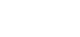where each
Xi is the span of the corresponding Jordan chain, and k is the number of Jordan chains.

One can also obtain a slightly different decomposition via the Jordan form. Given an eigenvalue λ
i, the size of its largest corresponding Jordan block si is called the index of λi and denoted by ν(λi). (Therefore the degree of the minimal polynomial is the sum of all indices.) Define a subspace Yi by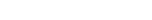This gives the decomposition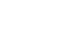where
l is the number of distinct eigenvalues of A. Intuitively, we glob together the Jordan block invariant subspaces corresponding to the same eigenvalue. In the extreme case where A is a multiple of the identity matrix we have k = n and l = 1.

The projection onto
Yi and along all the other Yj ( ji ) is called the spectral projection of A at λi and is usually denoted by Pi ; A). Spectral projections are mutually orthogonal in the sense that Pi ; A) Pj ; A) = 0 if ij. Also they commute with A and their sum is the identity matrix. Replacing every λi in the Jordan matrix J by one and zeroising all other entries gives Pi ; J), moreover if U J U -1 is the similarity transformation such that A = U J U -1 then Pi ; A) = U Pi ; J) U -1.

Comparing the two decompositions, notice that, in general, lk. When A is normal, the subspaces Xi's in the first decomposition are one-dimensional and mutually orthogonal. This is the spectral theorem
Spectral theorem
In mathematics, particularly linear algebra and functional analysis, the spectral theorem is any of a number of results about linear operators or about matrices. In broad terms the spectral theorem provides conditions under which an operator or a matrix can be diagonalized...

for normal operators. The second decomposition generalizes more easily for general compact operators on Banach spaces.

It might be of interest here to note some properties of the index, ν(λ). More generally, for a complex number λ, its index can be defined as the least non-negative integer ν(λ) such that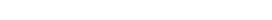So ν(λ) > 0 if and only if λ is an eigenvalue of A. In the finite dimensional case, ν(λ) ≤ the algebraic multiplicity of λ.

### Matrices with entries in a field

Jordan reduction can be extended to any square matrix M whose entries lie in a field
Field (mathematics)
In abstract algebra, a field is a commutative ring whose nonzero elements form a group under multiplication. As such it is an algebraic structure with notions of addition, subtraction, multiplication, and division, satisfying certain axioms...

K. The result states that any M can be written as a sum D + N where D is semisimple
Semisimple
In mathematics, the term semisimple is used in a number of related ways, within different subjects. The common theme is the idea of a decomposition into 'simple' parts, that fit together in the cleanest way...

, N is nilpotent
Nilpotent matrix
In linear algebra, a nilpotent matrix is a square matrix N such thatN^k = 0\,for some positive integer k. The smallest such k is sometimes called the degree of N....

, and DN = ND. This is called the Jordan–Chevalley decomposition. Whenever K contains the eigenvalues of M, in particular when K is algebraically closed, the normal form can be expressed explicitly as the direct sum
Direct sum
In mathematics, one can often define a direct sum of objectsalready known, giving a new one. This is generally the Cartesian product of the underlying sets , together with a suitably defined structure. More abstractly, the direct sum is often, but not always, the coproduct in the category in question...

of Jordan blocks.

Similar to the case when K is the complex numbers, knowing the dimensions of the kernels of (M − λI)k for 1 ≤ km, where m is the algebraic multiplicity of the eigenvalue λ, allows one to determine the Jordan form of M. We may view the underlying vector space V as a K[x]-module
Module (mathematics)
In abstract algebra, the concept of a module over a ring is a generalization of the notion of vector space, wherein the corresponding scalars are allowed to lie in an arbitrary ring...

by regarding the action of x on V as application of M and extending by K-linearity. Then the polynomials (x − λ)k are the elementary divisors of M, and the Jordan normal form is concerned with representing M in terms of blocks associated to the elementary divisors.

The proof of the Jordan normal form is usually carried out as an application to the ring
Ring (mathematics)
In mathematics, a ring is an algebraic structure consisting of a set together with two binary operations usually called addition and multiplication, where the set is an abelian group under addition and a semigroup under multiplication such that multiplication distributes over addition...

K[x] of the structure theorem for finitely generated modules over a principal ideal domain
Structure theorem for finitely generated modules over a principal ideal domain
In mathematics, in the field of abstract algebra, the structure theorem for finitely generated modules over a principal ideal domain is a generalization of the fundamental theorem of finitely generated abelian groups and roughly states that finitely generated modules can be uniquely decomposed in...

, of which it is a corollary.

### Compact operators

In a different direction, for compact operator
Compact operator
In functional analysis, a branch of mathematics, a compact operator is a linear operator L from a Banach space X to another Banach space Y, such that the image under L of any bounded subset of X is a relatively compact subset of Y...

s on a Banach space
Banach space
In mathematics, Banach spaces is the name for complete normed vector spaces, one of the central objects of study in functional analysis. A complete normed vector space is a vector space V with a norm ||·|| such that every Cauchy sequence in V has a limit in V In mathematics, Banach spaces is the...

, a result analogous to the Jordan normal form holds. One restricts to compact operators because every point x in the spectrum of a compact operator T, the only exception being when x is the limit point of the spectrum, is an eigenvalue. This is not true for bounded operators in general. To give some idea of this generalization, we first reformulate the Jordan decomposition in the language of functional analysis.

#### Holomorphic functional calculus

Let X be a Banach space, L(X) be the bounded operators on X, and σ(T) denote the spectrum
Spectrum (functional analysis)
In functional analysis, the concept of the spectrum of a bounded operator is a generalisation of the concept of eigenvalues for matrices. Specifically, a complex number λ is said to be in the spectrum of a bounded linear operator T if λI − T is not invertible, where I is the...

of TL(X). The holomorphic functional calculus
Holomorphic functional calculus
In mathematics, holomorphic functional calculus is functional calculus with holomorphic functions. That is to say, given a holomorphic function ƒ of a complex argument z and an operator T, the aim is to construct an operatorf\,...

is defined as follows:

Fix a bounded operator T. Consider the family Hol(T) of complex functions that is holomorphic on some open set G containing σ(T). Let Γ = {γi} be a finite collection of Jordan curves such that σ(T) lies in the inside of Γ, we define f(T) by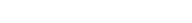The open set G could vary with f and need not be connected. The integral is defined as the limit of the Riemann sums, as in the scalar case. Although the integral makes sense for continuous f, we restrict to holomorphic functions to apply the machinery from classical function theory (e.g. the Cauchy integral formula). The assumption that σ(T) lie in the inside of Γ ensures f(T) is well defined; it does not depend on the choice of Γ. The functional calculus is the mapping Φ from Hol(T) to L(X) given by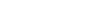We will require the following properties of this functional calculus:
1. Φ extends the polynomial functional calculus.
2. The spectral mapping theorem holds: σ(f(T)) = f(σ(T)).
3. Φ is an algebra homomorphism.

#### The finite dimensional case

In the finite dimensional case, σ(T) = {λi} is a finite discrete set in the complex plane. Let ei be the function that is 1 in some open neighborhood of λi and 0 elsewhere. By property 3 of the functional calculus, the operator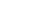is a projection. Moreoever, let νi be the index of λi and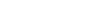The spectral mapping theorem tells us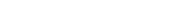has spectrum {0}. By property 1, f(T) can be directly computed in the Jordan form, and by inspection, we see that the operator f(T)ei(T) is the zero matrix.

By property 3, f(T) ei(T) = ei(T) f(T). So ei(T) is precisely the projection onto
the subspace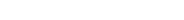The relation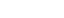implies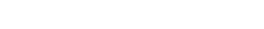where the index i runs through the distinct eigenvalues of T. This is exactly the invariant subspace decomposition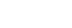given in a previous section. Each ei(T) is the projection onto the subspace spanned by the Jordan chains corresponding to λi.

This explicit identification of the operators ei(T) in turn gives an explicit form of holomorphic functional calculus for matrices:
For all f ∈ Hol(T),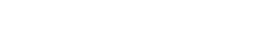Notice that the expression of f(T) is a finite sum because, on each neighborhood of λi, we have chosen the Taylor series expansion of f centered at λi.

#### Poles of an operator

Let T be a bounded operator λ be an isolated point of σ(T). (As stated above, when T is compact, every point in its spectrum is an isolated point, except possibly the limit point 0.)

The point λ is called a pole of operator T with order ν if the resolvent function RT defined by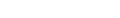has a pole of order ν at λ.

We will show that, in the finite dimensional case, the order of an eigenvalue coincides with its index. The result also holds for compact operators.

Consider the annular region A centered at the eigenvalue λ with sufficiently small radius ε such that the intersection of the open disc Bε(λ) and σ(T) is {λ}. The resolvent function RT is holomorphic on A.
Extending a result from classical function theory, RT has a Laurent series
Laurent series
In mathematics, the Laurent series of a complex function f is a representation of that function as a power series which includes terms of negative degree. It may be used to express complex functions in cases where...

representation on A: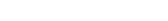where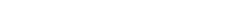and C is a small circle centered at λ.

By the previous discussion on the functional calculus,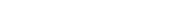where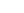is 1 on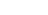and 0 elsewhere.

But we have shown that the smallest positive integer m such that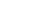and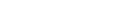is precisely the index of λ, ν(λ). In other words, the function RT has a pole of order ν(λ) at λ.

## Example

This example shows how to calculate the Jordan normal form of a given matrix. As the next section explains, it is important to do the computation exactly instead of rounding the results.

Consider the matrix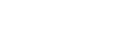which is mentioned in the beginning of the article.

The characteristic polynomial
Characteristic polynomial
In linear algebra, one associates a polynomial to every square matrix: its characteristic polynomial. This polynomial encodes several important properties of the matrix, most notably its eigenvalues, its determinant and its trace....

of A is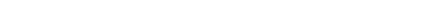This shows that the eigenvalues are 1, 2, 4 and 4, according to algebraic multiplicity. The eigenspace corresponding to the eigenvalue 1 can be found by solving the equation Av = λ v. It is spanned by the column vector v = (−1, 1, 0, 0)T. Similarly, the eigenspace corresponding to the eigenvalue 2 is spanned by w = (1, −1, 0, 1)T. Finally, the eigenspace corresponding to the eigenvalue 4 is also one-dimensional (even though this is a double eigenvalue) and is spanned by x = (1, 0, −1, 1)T. So, the geometric multiplicity (i.e. dimension of the eigenspace of the given eigenvalue) of each of the three eigenvalues is one. Therefore, the two eigenvalues equal to 4 correspond to a single Jordan block, and the Jordan normal form of the matrix A is the direct sum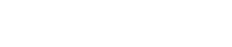There are three chains. Two have length one: {v} and {w}, corresponding to the eigenvalues 1 and 2, respectively. There is one chain of length two corresponding to the eigenvalue 4. To find this chain, calculate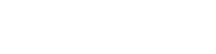Pick a vector in the above span that is not in the kernel of A − 4I, e.g., y = (1,0,0,0)T. Now, (A − 4I)y = x and (A − 4I)x = 0, so {y, x} is a chain of length two corresponding to the eigenvalue 4.

The transition matrix P such that P−1AP = J is formed by putting these vectors next to each other as follows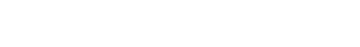A computation shows that the equation P−1AP = J indeed holds.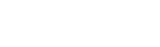If we had interchanged the order of which the chain vectors appeared, that is, changing the order of v, w and {x, y} together, the Jordan blocks would be interchanged. However, the Jordan forms are equivalent Jordan forms.

## Numerical analysis

If the matrix A has multiple eigenvalues, or is close to a matrix with multiple eigenvalues, then its Jordan normal form is very sensitive to perturbations. Consider for instance the matrix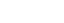If ε = 0, then the Jordan normal form is simply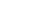However, for ε ≠ 0, the Jordan normal form is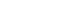This ill conditioning
Condition number
In the field of numerical analysis, the condition number of a function with respect to an argument measures the asymptotically worst case of how much the function can change in proportion to small changes in the argument...

makes it very hard to develop a robust numerical algorithm for the Jordan normal form, as the result depends critically on whether two eigenvalues are deemed to be equal. For this reason, the Jordan normal form is usually avoided in numerical analysis
Numerical analysis
Numerical analysis is the study of algorithms that use numerical approximation for the problems of mathematical analysis ....

; the stable Schur decomposition
Schur decomposition
In the mathematical discipline of linear algebra, the Schur decomposition or Schur triangulation, named after Issai Schur, is a matrix decomposition.- Statement :...

is often a better alternative.

## Powers

If n is a natural number
Natural number
In mathematics, the natural numbers are the ordinary whole numbers used for counting and ordering . These purposes are related to the linguistic notions of cardinal and ordinal numbers, respectively...

, the nth power of a matrix in Jordan normal form will be a direct sum of upper triangular matrices, as a result of block multiplication
Block matrix
In the mathematical discipline of matrix theory, a block matrix or a partitioned matrix is a matrix broken into sections called blocks. Looking at it another way, the matrix is written in terms of smaller matrices. We group the rows and columns into adjacent 'bunches'. A partition is the rectangle...

. More specifically, after exponentiation each Jordan block will be an upper triangular block.

For example,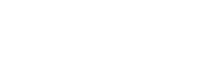Further, each triangular block will consist of λn on the main diagonal,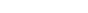times λn-1 on the upper diagonal, and so on. This expression is valid for negative integer powers as well if one extends the notion of the binomial coefficients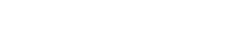.

For example,## See also

• Frobenius normal form
Frobenius normal form
In linear algebra, the Frobenius normal form, Turner binormal projective form or rational canonical form of a square matrix A is a canonical form for matrices that reflects the structure of the minimal polynomial of A and provides a means of detecting whether another matrix B is similar to A...

• Matrix decomposition
Matrix decomposition
In the mathematical discipline of linear algebra, a matrix decomposition is a factorization of a matrix into some canonical form. There are many different matrix decompositions; each finds use among a particular class of problems.- Example :...

• Canonical form
Canonical form
Generally, in mathematics, a canonical form of an object is a standard way of presenting that object....

• Jordan matrix
Jordan matrix
In the mathematical discipline of matrix theory, a Jordan block over a ring R is a matrix composed of 0 elements everywhere except for the diagonal, which is filled with a fixed element \lambda\in R, and for the superdiagonal, which is composed of ones...

The source of this article is wikipedia, the free encyclopedia.  The text of this article is licensed under the GFDL.